# Test: Flow Through Pipes - 2

## 10 Questions MCQ Test GATE Civil Engineering (CE) 2023 Mock Test Series | Test: Flow Through Pipes - 2

Description
Attempt Test: Flow Through Pipes - 2 | 10 questions in 30 minutes | Mock test for Civil Engineering (CE) preparation | Free important questions MCQ to study GATE Civil Engineering (CE) 2023 Mock Test Series for Civil Engineering (CE) Exam | Download free PDF with solutions
QUESTION: 1

### Three identical pipes of length L, diameter D and friction factor f, are connected in parallel between two reservoirs. The size of a pipe of length L and of same friction factor f, equivalent to the above pipes, is

Solution:

Equivalent pipe for the pipes in parallel: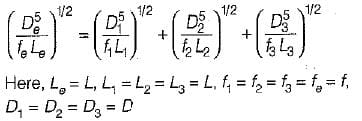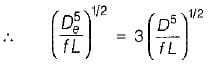or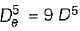or  De = 1.5518 D

QUESTION: 2

### A pipe of diameter D is to be replaced by n pipe each of diameter of laid in parallel. The value of d is given by

Solution: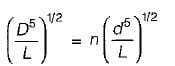or D5 = n2d5
or D = n2/5 D
∴ d = (D/n2/5)

QUESTION: 3

### At a sudden expansion in a horizontal pipe

Solution:
QUESTION: 4

In a pipeline, the H.G.L is above the pipe center line in the longitudinal section at point A and below the pipe center line at another point B.
From this it can be inferred that

Solution:
QUESTION: 5

In network of pipes

Solution:
QUESTION: 6

Consider the following statements:
1. Pipe network analysis is normally necessary in analyzing flow in pipes at city water systems,
2. Hardy-cross method of solving pipe network is a method of successive approximations and is not a direct method.
3. The network must satisfy the momentum equation because the flow in each pipe satisfies the head loss equation.
4. Principle of continuity is satisfied in a pipe network..

Select the correct statments:

Solution:
QUESTION: 7

The diameter of the nozzle (d) for maximum transmission of power is given by
Where
D = Diameter of pipe
f = Darcy’s friction factor
l = Length of pipe

Solution:

For maximum power transmission through nozzle:
Nozzle diameter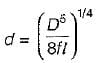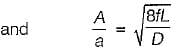A = area of supply pipe
a = area of Nozzle

QUESTION: 8

Maximum efficiency of transmission of power through a pipe is

Solution:

Efficiency of power transmission is given by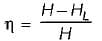For maximum efficiency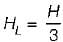We get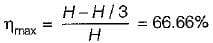QUESTION: 9

Power transmitted through a pipe is maximum when
Where
HL = head loss due to friction

Solution:

For maximum power transmission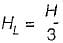QUESTION: 10

Power transmitted through a pipe is given by
where
w = specific weight of the fluid flowing through pipe
Q = discharge, m3/s

Solution:

Power transmitted through a pipe (When HL is frictional head)
P = wQ(H - HL)Use Code STAYHOME200 and get INR 200 additional OFF Use Coupon Code